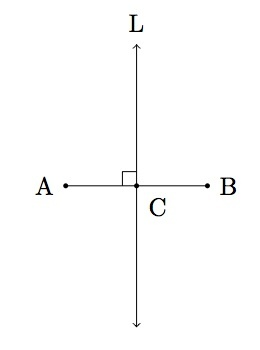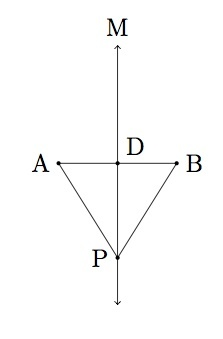# Points equidistant from two points in the plane

Alignments to Content Standards: G-CO.C.9

Suppose $A$ and $B$ are two distinct points in the plane and $L$ is the perpendicular bisector of segment $\overline{AB}$ as pictured below:1. If $C$ is a point on $L$, show that $C$ is equidistant from $A$ and $B$, that is show that $\overline{AC}$ and $\overline{BC}$ are congruent.
2. Conversely, show that if $P$ is a point which is equidistant from $A$ and $B$, then $P$ is on $L$.
3. Conclude that the perpendicular bisector of $\overline{AB}$ is exactly the set of points which are equidistant from $A$ and $B$.

## IM Commentary

This task is part of a series presenting important foundational geometric results and constructions which are fundamental for more elaborate arguments. They are presented without a real world context so as to see the important hypotheses and logical steps involved as clearly as possible. Further tasks, showing how these results can be used in context, will also be developed. Teachers should choose an appropriate mixture of abstract and contextual tasks which best fosters the development of their students' geometric intuition and understanding.

This task gives the important characterization of the perpendicular bisector of a line segment as the set of points equidistant from the endpoints of the segment. In the first part of the task, the instructor may need to suggest that there are two cases to consider:

• the case where $C$ is on the line segment $\overline{AB}$ (this is a special case and the students may forget to consider this)
• the case where $C$ is not on the line segment $\overline{AB}$.

The same two cases occur in part (b) for the point $P$.

The instructor may also wish to go over the logic of part (b) of the task and make sure that the students understand what needs to be shown and how it is different from part (a). Both (a) and (b) proceed via triangle congruence so the students need to be familiar with and confident in implementing these criteria. Because the task is relatively long and detailed it is recommended mainly for instructional purposes.

This task includes an experimental GeoGebra worksheet, with the intent that instructors might use it to more interactively demonstrate the relevant content material. The file should be considered a draft version, and feedback on it in the comment section is highly encouraged, both in terms of suggestions for improvement and for ideas on using it effectively. The file can be run via the free online application GeoGebra, or run locally if GeoGebra has been installed on a computer.

This file can be used individually to walk through the given set of problems. It can also be used in a presentation format for an audience. Each step has solutions which can be shown by clicking the check boxes that appear. The buttons are how you switch between steps and at any time you are able to drag the red point on the perpendicular bisector up and down.

## Attached Resources

• GeoGebra file
• ## Solution

1. We consider first the case where $C$ is not on segment $\overline{AB}$ as in the picture below. The point of intersection of $L$ and the segment $\overline{AB}$ is labelled $O$:By hypothesis, angles $COA$ and $COB$ are both right angles and so are congruent. Side $\overline{CO}$ is congruent to itself and side $\overline{OA}$ is congruent to side $\overline{OB}$ because $L$ bisects segment $\overline{AB}$. By SAS we conclude that triangle $COA$ is congruent to triangle $COB$. Thus segment $\overline{CA}$ is congruent to segment $\overline{CB}$ and so $C$ is equidistant from $A$ and $B$ as desired.

Next we consider the case where $C$ is on segment $\overline{AB}$ as pictured below:This case is simpler than the previous one because we know by hypothesis that $L$ bisects segment $\overline{AB}$ and so $\overline{CA}$ is congruent to $\overline{CB}$ and hence $C$ is equidistant from $A$ and $B$ here too.

2. Here we assume that $P$ is equidistant from $A$ and $B$. There are again two cases to consider. First, $P$ could be on segment $\overline{AB}$ and then $P$ must be the midpoint of segment $\overline{AB}$ and so $P$ is on $L$. Next suppose $P$ is not on line $L$. In this case, we let $D$ denote the midpoint of $\overline{AB}$ and $M$ the line joining $P$ and $D$ as pictured below:We know that $\overline{PA}$ is congruent to $\overline{PB}$ since $P$ is equidistant from $A$ and $B$ by assumption. We know that $\overline{DA}$ is congruent to $\overline{DB}$ because $D$ is the midpoint of $\overline{AB}$. Finally $\overline{PD}$ is congruent to $\overline{PD}$. Therefore by SSS, triangle $PDA$ is congruent to triangle $PDB$. Since angles $PDA$ and $PDB$ are congruent and add up to $180$ degrees, they must both be right angles. Since $\overline{DA}$ is congruent to $\overline{DB}$ we conclude that line $M$ is the perpendicular bisector of segment $\overline{AB}$, that is $M=L$.
3. In part (a) we saw that every point on the perpendicular bisector of segment $\overline{AB}$ is equidistant from points $A$ and $B$. In part (b) we saw that any point equidistant from points $A$ and $B$ is on the perpendicular bisector of segment $\overline{AB}$. Therefore the perpendicular bisector of $\overline{AB}$ is made up exactly of those points $P$ which are equidistant from $A$ and $B$.